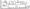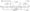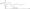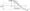# Control Systems July 2006

Note: 1. Answer any FIVE full questions,

1 a. Explain the difference between open loop and closed loop control systems, with one example for each.

b. For the mechanical system shown in figure Q1 (b),

i)  Write the differential equations governing the dynamic behaviour of the system.

ii)  Write an F-V analogous electric network.Fig. Ql(b)

c.Draw a block diagram representation for the electric circuit shown in figure Q1 (c) and evaluate the transfer function.

2 a.Define the term “transfer function”. The unit step response of single loop, unity feed back control system is given by, c(0 = l-1.25e‘2‘+0.25e’10‘Determine its closed loop and open loop transfer functions.

b.  Draw the signal flow graph for the block diagram shown in figure Q2 (b) and evaluate3 a. For the system shown in figure Q3 (a), CO)

i)       Identify the ‘Type’ of E(s)

ii)     Find the values of Kp, Kv and K*

ii)     If r(t) – 10 u(t), find the steady state value of the out put.

b.  Find the open loop transfer function of an equivalent prototype, single loop unity feed back system second order, whose step response is as shown in figure Q3 (b).Fig Q3 (b)

c. The open loop transfer function of a unity feed back, single loop control system is given by, Find the value of K so that the steady state error for a unit parabolic input is < 0.1 For the value of K found in part (i), verify whether the closed loop system is stable or otherwise.

4 a. For the characteristic equations given below determine the number of roots with positive real part.

i)     / + s5 + 3s4 + 2s3 + 5s2 + 3s +1 = 0

ii)  / + s7 + 4/ + 3s5 +14s4 +1 Is2 + 20 s2 + 95 + 9 = 0

b.  The open loop transfer function of a single loop, unity feed back control system is given by, = _£(£±13)_ sO + 3)C? + 7)

i)     Find the value of K for which the closed loop system is stable.

ii)   Find the value of K for which the closed loop poles are more negative than –

5 a. For a single loop, unity feed back system, the open loop transfer function is given by, *(J + 2)(, + 3) s(s + Y) Show that the complex part of the root locus is a circle. Identify its centre and radius.

b.  The characteristic equation of a single loop unity feed back control system is given by,(s1) ~ s3 + 8s2 + 20s + k — 0 Sketch the complete root locus diagram and from that find,

i)              Two values of K that make the system critically damped

ii)             Two values of K for which the damping ratio is 0.95.

iii)            Write closed loop transfer functions for the values of K found in part (ii).

6 a. For the loop gain function given below, determine the value of K analytically, so that the gain margin is 12 dB. GH(s) =s(j2 +13j + 121) Further, for this value of K, find analytically (without plotting Bode plot), the phase margin.

b.  The open loop transfer function of a prototype single loop, unity feed back control system is given by, G(s) =s(s + 2 Sa>n) Show that the phase margin is given by,K PM = tan-‘l.2S^2S2 +-sjl + (2S2f

c.   Suppose that G(s) in part (b) is modified to read, G(s) = – ,y(l + sr)

i)   Determine the values of K and x, so that Mr – 1.06 and con = 12rad/sec,

ii)   For the values of K and t so found, evaluate the corresponding 3 dB bandwidth.

7 a.  Find the open loop transfer function of a single loop unity feed back system whose asymptotic Bode magnitudeplotis as shown in figure Q7 (a).b. The open loop transfer function of a unity feed back single loop control system is given by, G(s) =(1 + 0.02s)(1 + 0,05j)

C. Draw the asymptotic Bode plots and hence find the value of K for which the gain margin is 10 dB. What is the corresponding phase margin?

8  a. State and explain Nyquist stability criterion.

b.  The open loop transfer function of a single loop unity feed back system is given by, = A^lXs + 3) s(s + 2)(s – 4) Draw the complete Nyquist diagram and there from find the range of K for which the system is absolutely stable. Verify your answer using R-H criterion.